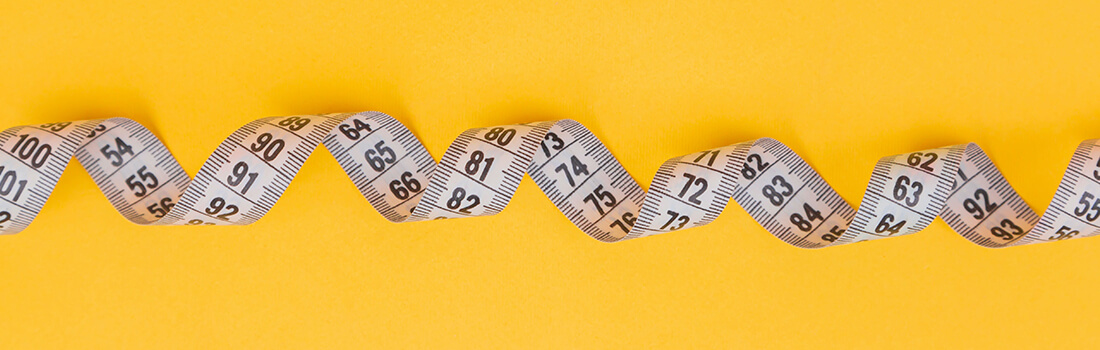# Length conversion calculator | Length unit conversion

Our free length conversion calculator offers conversion between the most common units of length. You can convert mm, cm, dm, m, km, in, ft, yd, mi through the converter on this website.

Millimeters (mm)
Centimeters (cm)
Decimeters (dm)
Meters (m)
Kilometers (km)
Inches (in)
Feet (ft)
Yards (yd)
Miles (mi)

## How to use length conversion calculator?

### ~ Step 1 ~

Choose the unit of length that you would want to convert.

### ~ Step 2 ~

Enter the length (It has to be a number).

### ~ Step 3 ~

Length conversion calculator will generate all measurement conversions at once.## Units of length

### Millimeter

A millimeter (mm) is 1/1000 of a meter. Latin word Milli can be translated as thousand.

### Centimeter

A centimeter (cm) equals 1/100 of a meter. 1 cm is equivalent to 0,01 m.

### Meter

A meter (m) is the fundamental unit of length in the International system of units.

### Inch

An inch (in) unit of length in the Imperial system. The size of the inch is 2,54 cm.

### Foot

A foot is (ft) a unit of length in the imperial system of units that equals to 12 inches.

### Yard

A yard (yd) is the standard unit of length in the USA or Canada that equals to 3 feet or 36 inches.

### Kilometer

A kilometer (km) is a unit of distance that equals to 1000 meters. 1 km = 0,62 mile.

### Mile

A mile (mi) is a British Imperial unit of distance. A Mile is equivalent to 5280 feet.

### Decimeter

A decimeter is 1/10 of a meter which is derived from the word DECI.

## Length conversion chart. How to do calculations

Many people work daily with units of length. We talk about Engineers, Fashion Designers, Construction Workers, or Architects. These conversion charts and our length conversion calculator are the best way to understand how to make the conversion from one unit of length to another.

### ~ The metric system is based on succession. Each unit is 10 times larger than the previous unit. ~

1 kilometre = 10 Hectometres = 1 Decametres = 10 Metres = 100 Decimetres = 1000 Centimeters = 10000 Millimeters

### ~ US customary units can be calculated based on this pattern. ~

1 mile = 1760 yards = 5280 feet = 63360 inches

## The Imperial System of Units and International System of Units

There are 2 main systems of measurement. International System of Units aka Metric and Imperial System of Units. While US customary and Imperial units are mostly used in the United Kingdom and USA, the International system of units has spread around the world, mainly in non-English speaking countries. For example, metric units of length are commonly used by Europeans.

## What are the units of length from smallest to largest?

The Metric system is based on the following units of length. The smallest unit is on the left, largest on the right.

millimeter, centimeter, decimeter, meter, decameter, hectometer, kilometer

### In the British Imperial system are units of length ordered from smallest to largest like this:

inch, foot, yard, rod, furlong, mile, league